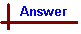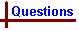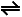More Acids and Bases 1 Link to Data Page Your feedback on these self-help problems is appreciated. Click here to send an e-mail. For on-line help on this topic, please see the following Chemcal modules: Acids and Bases (strong acids and bases; conjugate acid/base pairs; pH;self-dissociation of water) Weak Acids and Bases (weak acids; weak bases; Ka and Kb) Calculations with Weak Acids and Bases (calculations involving weak acids and bases and buffers) Acid-base Titrations (titration curves for acid/base titrations; indicators) Shortcut to Questions Q: 1 2 3 4 5 6 7 8 9 10 11 1 There is a reaction between an acid and a base when a compound containing hydrated aluminium ions are added to water. (1.) How can this be shown experimentally? (2.) Write the formula of the reacting acid. (3.) Write the formula of the reacting base.2 Predict whether an aqueous solution of the following salts will be acidic, neutral or basic: (1.) Na3PO4 (2.) Na2HPO4 (3.) NaH2PO43 Predict whether 0.01 M solutions of the following substances will be acidic, neutral or basic: (1.) rubidium iodide (2.) iron(II) bromide (3.) ammonium dihydrogenphosphate (4.) lithium acetate (5.) potassium hydrogenphosphate (6.) ammonium chloride (7.) copper(II) nitrate (8.) magnesium nitrate (9.) barium hydroxide4 One mole of each of the following is added separately to one litre of pure water. What will the pH of the solution be? Write >7, =7 or <7. (1.) CsF (2.) FeSO4 (3.) FeCl3 (4.) BaS (5.) KCl (6.) BaI2 (7.) Al2Cl65 The hydrides of the elements listed below are added to separate samples of water. Will any solution which results be acidic, neutral or basic? Write the equation for the dissolution of each hydride in water, showing the ionic species formed. (1.) N (2.) Br (3.) S6 What mass of ammonium chloride must be dissolved in water (200 cm3) to give a solution whose pH is 4.75?7 (1.) A water solution contains ammonium chloride (2.34 g) in 250 cm3 of solution and has a pH of 5.00. Use this information to calculate KA for NH4+. (2.) Using the value of KA for NH4+ calculated in (1.), calculate KB for ammonia.8 What is the pH of a water solution made by dissolving sodium ethoxide (34 g) and making the volume up to 1.00 litre?9 Give the pH of aqueous solutions prepared from equimolar amounts of the following pairs of substances: (1.) H3PO4 / NaH2PO4 (2.) NaH2PO4 / Na2HPO4 (3.) Na2HPO4 / Na3PO410 Calculate the - (1.) pH of 0.10 M acetic acid (2.) pH of a solution 1.0 M with regard to both acetic acid and sodium acetate (3.) pH of a solution 0.10 M with regard to both acetic acid and sodium acetate11 Calculate the ratio [CH3COOH] : [CH3CO2-] in acetic acid - acetate solutions with the following pH values: (1.) 2.00 (2.) 3.00 (3.) 5.00 (4.) 7.00 (5.) 9.00More Acids and Bases 1 (Answers) 1 (1.) An acid - base reaction with water will involve a change in [H3O+], so it can be shown experimentally by noting a pH change upon addition of the aluminium salt (such as Al(NO3)3, where the anion does not hydrolyse in water.) (2.) The reacting acid is [Al(H2O)6]3+ (ie this is the proton donor) (3.) The reacting base is water, H2O (ie water accepts a proton to become H3O+)2 (1.) PO43- cannot act as an acid as it has no H atoms in its structure. Its conjugate acid is a weak acid, therefore PO43- is a weak base and its water solution is basic. PO43- + H2OOH- + HPO42- (2.) HPO42- is amphiprotic (can act as either acid or base). In water solution: HPO42- + H2OH3O+ + PO43- (Eqn 1, KA = 10-12.36) HPO42- + H2OOH- + H2PO4- (Eqn 2, KB = 10-1.64) As KB (HPO42-) > KA (HPO42-), Eqn 2 predominates and the solution will have a pH > 7. (3.) H2PO4- is amphiprotic (H3PO4 is it's conjugate acid, HPO4- it's conjugate base) In water solution: H2PO4- + H2OHPO42- + H3O+ (Eqn 1, KA = 10-7.20) H2PO4- + H2OH3PO4 + OH- (Eqn 2, KB = 10-6.80) As KB (H2PO42-) > KA (H2PO42-), Eqn 2 predominates and the solution will have a pH > 7.3 These questions can be approached by looking at whether either the cation or anion participate in an acid-base reaction with the solvent, water, and then considering whether any reaction occurring produces an acidic or a basic solution. The following should be remembered: Most hydrated metal cations behave as acids in water. Those that are not acids are metal cations with a +1 charge (M+), and a few of the larger M2+ ions (Ba2+, Sr2+, Ca2+). Other hydrated metal cations behave as acids in water due to the loss of one of the H+ ions from water molecules attached to the cation. Anions that are not basic in water are the conjugate bases of strong acids (Cl-, Br-, I-, NO3-, ClO4-). Any anion that is the conjugate base of a weak acid will itself be a weak base. Solutions containing only ions from these two groups will be neutral, since no acid-base reaction with water takes place. The presence of any other cation or anion might lead to an acidic or basic solution. (1.) RbI. Neither Rb+ nor I- enter into acid-base reactions with water, so the solution is neutral. Rb+ is not acidic due to it's low charge density. I- is not a base in water as it's conjugate acid HI is a strong acid, so the reaction I- + H2OHI + OH- cannot occur. (2.) FeBr2. Br- does not hydrolyse water, so it has no effect on the pH. Fe2+ exists in solution as the hydrated species Fe(H2O)62+. The positively charged Fe ion can draw enough electron density from the bound water H-O bonds to release a proton: Fe(H2O)62+ + H2OFe(H2O)5OH+ + H3O+ Many small and highly charged metal cations are capable of dissociating in water this way, leading to acidic solutions. (3.) NH4H2PO4. In this case both NH4+ and H2PO4- could enter into acid-base reactions with water and contribute to the pH of the solution, so KA (or pKA) values need to be considered. NH4+ is a weak acid, and the two possible reactions are: NH4+ + H2OH3O+ + NH3 pKA of NH4+ = 9.24. ∴ KA = 5.8 x 10-10 H2PO4- + H2OHPO42- + H3O+ H2PO4- is amphiprotic, but its behaviour as a weak acid predominates (see previous question). ∴ Both cation and anion behave as acids, and the solution will be acidic. (4.) CH3CO2Li. Dissociation in water gives lithium and acetate ions. Li+ does not behave as an acid in water, so it has no effect on the pH. CH3CO2- is the conjugate base of acetic acid, CH3COOH, a weak acid, so the following equilibrium is set up: CH3CO2- + H2OCH3COOH + OH- As a result, the solution is basic due to production of OH- ions. (5.) Basic: HPO42- + H2OH2PO4- + OH-, the K+ cation has no effect on pH. (6.) Acidic: NH4+ + H2ONH3 + H3O+, the Cl- anion has no effect on pH. (7.) Acidic: [Cu(H2O)4]2+ + H2O[Cu(H2O)3OH]+ + H3O+, the NO3- anion has no effect on pH. (8.) Acidic: [Mg(H2O)6]2+ + H2O[Mg(H2O)5OH]+ + H3O+, the NO3- anion has no effect on pH. (9.) Basic: Barium hydroxide is soluble, releasing Ba2+ and OH-ions in solution. Ba(OH)2 + H2O → Ba2+ + 2OH-, the Ba2+ is one of the large 2+ charged metal cations which do not react with water and so have no effect on pH.4 (1.) F- + H2OHF + OH- (pH > 7) (2.) [Fe(H2O)6]2+ + H2O[Fe(H2O)5OH]+ + H3O+ (pH < 7) (3.) [Fe(H2O)6]3+ + H2O[Fe(H2O)5OH]2+ + H3O+ (pH < 7) (4.) S2- + H2OHS- + OH- (pH > 7) (5.) Neither K+ nor Cl- react with water (pH = 7) (6.) Neither Ba2+ nor I- react with water (pH = 7) (7.) [Al(H2O)6]3+ + H2O[Al(H2O)5OH]2+ + H3O+ (pH < 7)5 (1.) The hydride of nitrogen is ammonia, NH3. Ammonia dissolves in water and causes hydrolysis to form a weakly basic solution: NH3 + H2ONH4+ + OH-. (2.) The hydride of bromine is hydrogen bromide, HBr. Hydrogen bromide dissolves in water and completely ionises to form an acidic solution: HBr + H2OH3O+ + Br- (3.) The hydride of sulfur is hydrogen sulfide, H2S. Hydrogen sulfide dissolves in water and dissociates to a small extent to form an acidic solution. H2S is a diprotic acid, the first dissociation forms HS-, the second forms S2- (to a much smaller degree) H2S + H2OH3O+ + HS- HS- + H2OH3O+ + S2-6 NH4+ + H2OH3O+ + NH3 The [H3O+] for a pH of 4.75 is 10-4.75 = 1.78 x 10-5 mol L-1 KA = [H3O+] [NH3] / [NH4+] KA = 10(-pKA) = 10-9.24 From the stoichiometry of the reaction, [H3O+] = [NH3] ∴ KA = [H3O+]2 / [NH4+] [NH4+] = [H3O+]2 / KA = (1.78 x 10-5 ) / (10-9.24) = 0.551 mol L-1 No. mol NH4Cl required = volume of solution x [NH4+] = 0.200 L x 0.551 mol L-1 = 0.110 mol. Mass of NH4Cl required = molar mass NH4Cl x no. mol NH4Cl = 53.49 x 0.110 = 5.9 g.7 (1.) [NH4+]init = 0.175 mol L-1 [H3O+]eqb = 10-5.00 = 1.0 x 10-5 mol L-1 NH4+ + H2OH3O+ + NH3 KA = [H3O+] [NH3] / [NH4+] From the stoichiometry of the reaction, [H3O+] = [NH3] ∴ KA = [H3O+]2 / [NH4+] [NH4+]init = [NH4+]eqb + 1.0 x 10-5 » 1.0 x 10-5 ∴ KA = [H3O+]2 / [NH4+]init = (1.0 x 10-5 / 0.175 = 5.7 x 10-10 (2.) From KA(NH4+) x KB(NH3) = KW = 1.0 x 10-14 KB(NH3) = (1.0 x 10-14 ) / (5.7 x 10-10 ) = 1.8 x 10-5 .8 CH3CH2O- + H2O → OH- + CH3CH2OH CH3CH2O- is a stronger base than OH-, so reaction goes to completion. There are 0.500 mol CH3CH2ONa in 1 L ∴ [OH-] = 0.500 mol L-1 (from above equation) Calculating [H3O+] for pH: [H3O+] = 1.0 x 10-14 / 0.500 = 2.0 x 10-14 mol L-1 ∴ pH = -log (2.0 x 10-14) = 13.709 KA = [H3O+] [A-] / [HA] Now in the case of a buffer, [H3O+] does not equal [A-] because of the presence of additional conjugate base. Rather, [H3O+] = KA [HA] / [A-], showing that the [H3O+] is determined by (i) the Ka of the weak acid and (ii) the ratio of weak acid to weak base in the buffer. If the acid / conjugate base pair are present in equimolar quantities (ie [HA] = [A-]), they cancel out, leaving [H3O+] = KA. It then follows that pH = pKA. (1.) The conjugate acid / base pair in this system is H3PO4 / H2PO4-. They are present in equimolar quantities, so from the above working pH = pKA of H3PO4 = 2.13, and so pH of the buffer = 2.13. Note here that the pKA value used here must be that of the weak acid in the acid / base pair (ie H3PO4). Do not confuse with pKA of H2PO4-! (2.) The conjugate acid / base pair in this system is H2PO4- / HPO42-. They are present in equimolar quantities, so from the above working pH = pKA of H2PO4- = 7.20, and so pH of the buffer = 7.20. (3.) The conjugate acid / base pair in this system is HPO42- / PO43-. They are present in equimolar quantities, so from the above working pH = pKA of HPO42- = 12.36, and so pH of the buffer = 12.36.10 (1.) CH3COOH + H2OCH3CO2- + H3O+ [CH3COOH]init = 0.10 mol L-1 KA = [H3O+] [CH3CO2-] / [CH3COOH] = 10-pKa = 10-4.76 [H3O+] = [CH3CO2-] ∴ KA = [H3O+]2 / [CH3COOH]init [H3O+] = (KA x [CH3COOH])½ = (10-4.76 x 0.10 )½ = 1.32 x 10-3 mol L-1 pH = -log [H3O+] = 2.88 (2.) KA = [H3O+] [A-] / [HA] The acid (CH3COOH) and it's conjugate base (CH3CO2-) are present in equimolar amounts, so their respective terms cancel out, leaving KA = [H3O+] ∴ pH = pKA (CH3COOH) = 4.76 (3.) As for part (2.), the acid and it's conjugate base are present in equimolar amounts, so pH = pKA (CH3COOH) = 4.76 Note that the pH is determined not by the concentration of acid and base, but by the ratio of concentrations, so the 0.1 M and 1.0 M buffer solutions have the same pH. However, a very important property which does depend on the concentration of the buffer is the buffer capacity. Both the concentrated (1.0 M) and dilute (0.1 M) buffer solutions have the same pH when originally made up, but the more concentrated buffer solution will undergo less change in pH with added base or acid than the more dilute buffer - ie it has a greater capacity to withstand changes in added H3O+ or OH-.11 The acid / base ratio is found from KA: KA = [H3O+] [A-] / [HA] ∴ [HA] / [A-] = [H+] / KA The [H+] can be obtained from the quoted pH values: [H+] = 10-pH Substituting into the previous equation we obtain [HA] / [A-] = [H+] / KA ∴ [CH3COOH] / [CH3COO-] = [H+] / KA From the data source, KA for CH3COOH = 10-4.76 A worked example is provided below: (1.) pH = 2 [CH3COOH] / [CH3COO-] = 10-2.00 / 10-4.76 = 5.8 x 102 : 1 (2.) pH = 3.00, [CH3COOH] / [CH3COO-] = 5.8 x 101 : 1 (3.) pH = 5.00, [CH3COOH] / [CH3COO-] = 5.8 x 10-1 : 1 (4.) pH = 7.00, [CH3COOH] / [CH3COO-] = 5.8 x 10-3 : 1 (5.) pH = 9.00, [CH3COOH] / [CH3COO-] = 5.8 x 10-5 : 1